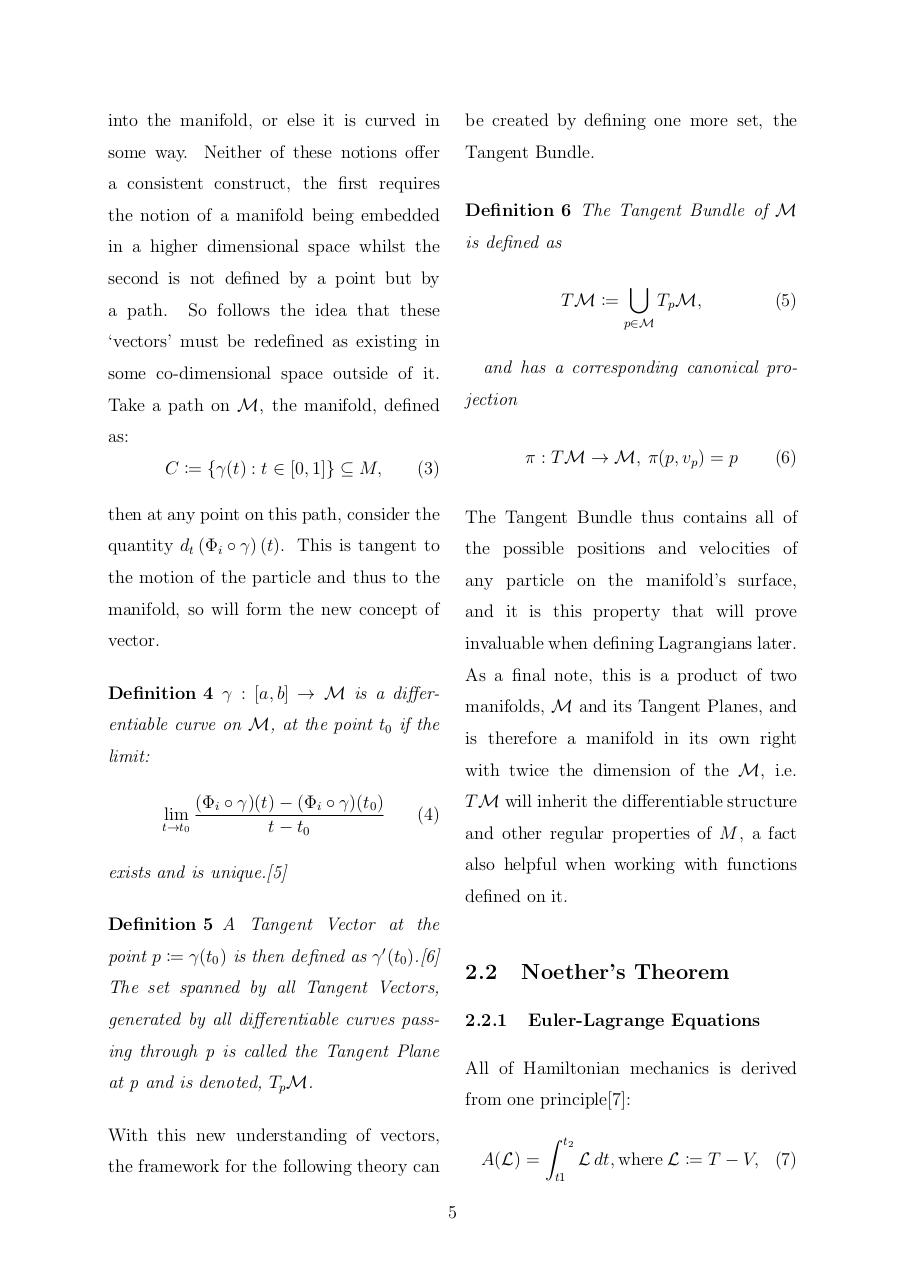# Noether's Theorem on Non Trivial Manifolds By Daniel Martin.pdfPage 1...3 4 56720

#### Text preview

into the manifold, or else it is curved in

be created by defining one more set, the

some way. Neither of these notions offer

Tangent Bundle.

a consistent construct, the first requires
the notion of a manifold being embedded

Definition 6 The Tangent Bundle of M

in a higher dimensional space whilst the

is defined as

second is not defined by a point but by
a path.

T M :=

So follows the idea that these

[

Tp M,

(5)

p∈M

‘vectors’ must be redefined as existing in
and has a corresponding canonical pro-

some co-dimensional space outside of it.

jection

Take a path on M, the manifold, defined
as:
C := {γ(t) : t ∈ [0, 1]} ⊆ M,

π : T M → M, π(p, vp ) = p

(3)

(6)

then at any point on this path, consider the

The Tangent Bundle thus contains all of

quantity dt (Φi ◦ γ) (t). This is tangent to

the possible positions and velocities of

the motion of the particle and thus to the

any particle on the manifold’s surface,

manifold, so will form the new concept of

and it is this property that will prove

vector.

invaluable when defining Lagrangians later.
As a final note, this is a product of two

Definition 4 γ : [a, b] → M is a differ-

manifolds, M and its Tangent Planes, and

entiable curve on M, at the point t0 if the

is therefore a manifold in its own right

limit:

with twice the dimension of the M, i.e.
lim

t→t0

(Φi ◦ γ)(t) − (Φi ◦ γ)(t0 )
t − t0

T M will inherit the differentiable structure

(4)

and other regular properties of M , a fact
also helpful when working with functions

exists and is unique.

defined on it.
Definition 5 A Tangent Vector at the
point p := γ(t0 ) is then defined as γ 0 (t0 ).

2.2

The set spanned by all Tangent Vectors,
generated by all differentiable curves pass-

2.2.1

Noether’s Theorem
Euler-Lagrange Equations

ing through p is called the Tangent Plane
All of Hamiltonian mechanics is derived

at p and is denoted, Tp M.

from one principle:

With this new understanding of vectors,

Z

t2

L dt, where L := T − V, (7)

A(L) =

the framework for the following theory can

t1

5